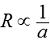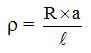Ex﻿

# 1. Electricity

#### Resistivity

ResistivitySo,or...(i)
where ρ (rho) is called resistivity of the material of conductor.
यदि, l = 1 m और a = 1 m2
तब R = ρ ...(ii)
Thus, if we take 1 metre long piece of a substance having a cross-sectional area of 1 meter2, then the resistance of that piece of the substance is called its resistivity.
Resistivity of a substance can also be defined as follows :
The resistance offered by a cube of a substance having side of 1 metre, when current flows perpendicular to the opposite faces, is called its resistivity.
Units of Resistivity
From equation (i), we can writeSo, SI unit of resistivity (ρ)
== ohm.m
Thus, the SI unit of resistivity is ohm. m (or Ω .m).

If you want to give information about online courses to other students, then share it with more and more on Facebook, Twitter, Google Plus. The more the shares will be, the more students will benefit. The share buttons are given below for your convenience.
×

#### NTSE Physics (Class X)

• Electricity
• Magnetic Effect of Curent
• Light Reflection
• Light Refraction
• Human Eye & Colourful World
• Source of Energy
• Motion

#### NTSE Physics (Class IX)

• Force & laws of motion
• Work and Energy
• Gravitation
• Sound

SHOW CHAPTERS